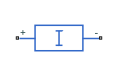Constant current load for DC or AC supply

Since R2021a

•Libraries:
Simscape / Electrical / Passive

## Description

The Constant Current Load block implements a constant current load for a DC or AC supply.

If you set the Load type parameter to `DC`:

• The consumed current of this block is equal to the value of the Consumed current parameter as long as the voltage from the DC supply is equal to or greater than the value specified for the Minimum supply voltage parameter.

• When the voltage from the DC supply drops below Minimum supply voltage, the load behaviour changes and the block models a resistive load. If the supply voltage becomes negative, the block models a small open-circuit conductance.

To ensure smooth transitions between these behaviours, the block uses a third-order polynomial spline with continuous derivatives. You can specify the width of this transition using the Transition voltage width parameter.

If you set the Load type parameter to `AC`:

• The root mean square consumed current of this block is equal to the value of the Consumed current (RMS) parameter as long as the voltage from the AC supply is equal to or greater than the value specified for the Minimum supply voltage (RMS) parameter.

• When the voltage from the AC supply drops below the Minimum supply voltage (RMS) parameter, the load behaviour changes and the block models a load with constant resistance.

### Equations

If you set the Load type parameter to `AC`, the block calculates the peak voltage, Vpk, through harmonic approximation of the instantaneous voltage by using a one-period-integrated Fourier transform:

`$\begin{array}{l}\mathrm{Re}={f}_{0}\int v\text{\hspace{0.17em}}sin\left(2\pi {f}_{0}t\right)\\ \mathrm{Im}={f}_{0}\int v\text{\hspace{0.17em}}sin\left(2\pi {f}_{0}t+\frac{\pi }{2}\right)\\ {V}_{pk}=2\sqrt{{\mathrm{Re}}^{2}+I{m}^{2}}\end{array}$`

During the first period, the peak voltage is equal to `0` `V`. The current is defined by this equation:

`$i\left(t\right)=\frac{V\left(t\right)}{{R}_{equiv}},$`

where Requiv is the equivalent resistance and depends on the value of the Minimum supply voltage (RMS) parameter.

If the voltage from the three-phase supply is greater than the value specified for the Minimum supply voltage (RMS) parameter, the equivalent resistance is defined by:

`${R}_{equiv}=\frac{\frac{{V}_{pk}}{\sqrt{2}}}{{I}_{RMS}{}_{consumed}},$`

where Vpk is the voltage peak magnitude and IRMSconsumed is the value of the Consumed current (RMS) parameter.

If the voltage from the three-phase supply is less than the value specified for the Minimum supply voltage (RMS) parameter, the equivalent resistance is defined by:

`${R}_{equiv}=\frac{{V}_{RMS}{}_{min}}{{I}_{RMS}{}_{consumed}},$`

where VRMSmin is the value of the Minimum supply voltage (RMS) parameter.

### Faults

The Constant Current Load block allows you to model an electrical fault as an open circuit. The block can trigger fault events either:

• At a specific time

• When a power limit is exceeded for longer than a specific time interval

You can also choose whether to issue an assertion when a fault occurs by using the Reporting when a fault occurs parameter. The assertion can take the form of a warning or an error. By default, the block does not issue an assertion.

If the block is in a network that is compatible with the frequency-time simulation mode, you can perform a load-flow analysis on the network. A load-flow analysis provides steady-state values that you can use to initialize a machine.

For more information, see Perform a Load-Flow Analysis Using Simscape Electrical and Frequency and Time Simulation Mode.

## Ports

### Conserving

expand all

Electrical conserving port associated with the constant current load positive terminal.

Electrical conserving port associated with the constant current load negative terminal.

## Parameters

expand all

### Main

Type of load. Select `DC` to use this load with a DC supply or `AC` to use this load with an AC supply.

Current consumed by the block.

#### Dependencies

To enable this parameter, set Load Type to `DC`.

Minimum voltage the DC supply needs to give the block to act as a constant current load.

#### Dependencies

To enable this parameter, set Load Type to `DC`.

Value of the reverse conductance when the supply voltage becomes negative.

#### Dependencies

To enable this parameter, set Load Type to `DC`.

Transition voltage width used to ensure smooth transitions between block behaviours when the supply voltage drops below the value of the Minimum supply voltage parameter or becomes negative.

#### Dependencies

To enable this parameter, set Load Type to `DC`.

Root mean square current consumed by the block.

#### Dependencies

To enable this parameter, set Load Type to `AC`.

Rated frequency.

#### Dependencies

To enable this parameter, set Load Type to `AC`.

Minimum voltage, in RMS, that the AC supply must provide for the block to act as a constant current load.

#### Dependencies

To enable this parameter, set Load Type to `AC`.

### Faults

Select `On` to enable faults modeling and expose parameters that allow you to select the reporting method and specify the trigger mechanism.

Conductance during the open-circuit state.

#### Dependencies

To enable this parameter, set Enable open-circuit fault to `On`.

Choose whether to issue an assertion when a fault occurs:

• `None` — The block does not issue an assertion.

• `Warn` — The block issues a warning.

• `Error` — Simulation stops with an error.

#### Dependencies

To enable this parameter, set Enable open-circuit fault to `On`.

Whether to trigger the fault based on temporal or behavioral conditions.

#### Dependencies

To enable this parameter, set Enable open-circuit fault to `On`.

Simulation time when the block enters the faulted state.

#### Dependencies

To enable this parameter, set Enable open-circuit fault to `On` and Fault trigger to `Temporal`.

Maximum permissible power value. If the power exceeds this value for longer than the Time to fail when exceeding power parameter value, then the block enters the faulted state.

#### Dependencies

To enable this parameter, set Enable open-circuit fault to `On` and Fault trigger to `Behavioral`.

Maximum length of time that the power can exceed the maximum permissible value without triggering the fault.

#### Dependencies

To enable this parameter, set Enable open-circuit fault to `On` and Fault trigger to `Behavioral`.

## Version History

Introduced in R2021a